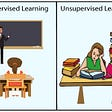# Machine Learning Notes

• Expectation-maximization (EM): assumes random components and computes for each point a probability of being generated by each component of the model. Then iteratively tweaks the parameters to maximize the likelihood of the data given those assignments. Example: Gaussian Mixture
• Gradient Boosting: optimization of arbitrary differentiable loss functions. — Risk of overfitting
• Hypothesis tests
• KNN: + Simple, flexible, naturally handles multiple classes. — Slow at scale, sensitive to feature scaling and irrelevant features
• K-means: aims to choose centroids that minimize the inertia, or within-cluster sum-of-squares criterion. Use the “elbow” method to identify the right number of means
• Lasso: linear model regularization technique with tendency to prefer solutions with fewer non-zero coefficients
• Linear Discriminant Analysis (LDA): A classifier with a linear decision boundary, generated by fitting class conditional densities to the data and using Bayes’ rule. The model fits a Gaussian density to each class, assuming that all classes share the same covariance matrix.
• Linear regression assumptions (LINE): 1) Linearity, 2) Independence of errors, 3) Normality of errors, 4) Equal variances. Tests of assumptions: i) plot each feature on x-axis vs y_error, ii) plot y_predicted on x-axis vs y_error, iii) histogram of errors
• Overfitting, bias-variance and learning curves. Overfitting (high variance) options: more data, increase regularization, or decrease model complexity
• Overspecified model: can be used for prediction of the label, but should not be used to ascribe the effect of a feature on the label
• PCA: transform data using k vectors that minimize the perpendicular distance to points. PCA can be also thought of as an eigenvalue/engenvector decomposition
• Receiver operating characteristic (ROC): relates true positive rate (y-axis) and false positive rate (x-axis). A confusion matrix defines TPR = TP / (TP + FN) and FPR = FP / (FP + TN)
• Preprocessing: duplicates -> outliers -> missing values -> feature correlation -> feature distribution/skew
• Naive Bayes
• Normal Equation
• Random Forests: each tree is built using a sample of rows (with replacement) from training set. + Less prone to overfitting
• Reinforcement Learning
• Ridge Regression regularization: imposes a penalty on the size of the coefficients
• R2: strength of a linear relationship. Could be 0 for nonlinear relationships. Never worsens with more features
• Sample variance: divided by n-1 to achieve an unbiased estimator because 1 degree of freedom is used to estimate b0
• Sigmoid
• SMOTE algorithm is parameterized with k_neighbors. Generate and place a new point on the vector between a minority class point and one of its nearest neighbors, located [0, 1] percent of the way from the original point
• SVM: Effective in high dimensional spaces (or when number of dimensions > number of examples). SVMs do not directly provide probability estimates
• Stochastic gradient descent cost function

--

--

--

Love podcasts or audiobooks? Learn on the go with our new app.

## How To Connect Python to Google Cloud’s Text-To-Speech## Basics of Deep Learning: Backpropagation## Part 5: Stock Market Latent Time Shifting with AutoEncoders## Deep Learning: Autocorrect, Spell check for short words## Trying to Explain regularization Techniques in Machine Learning as easy as I can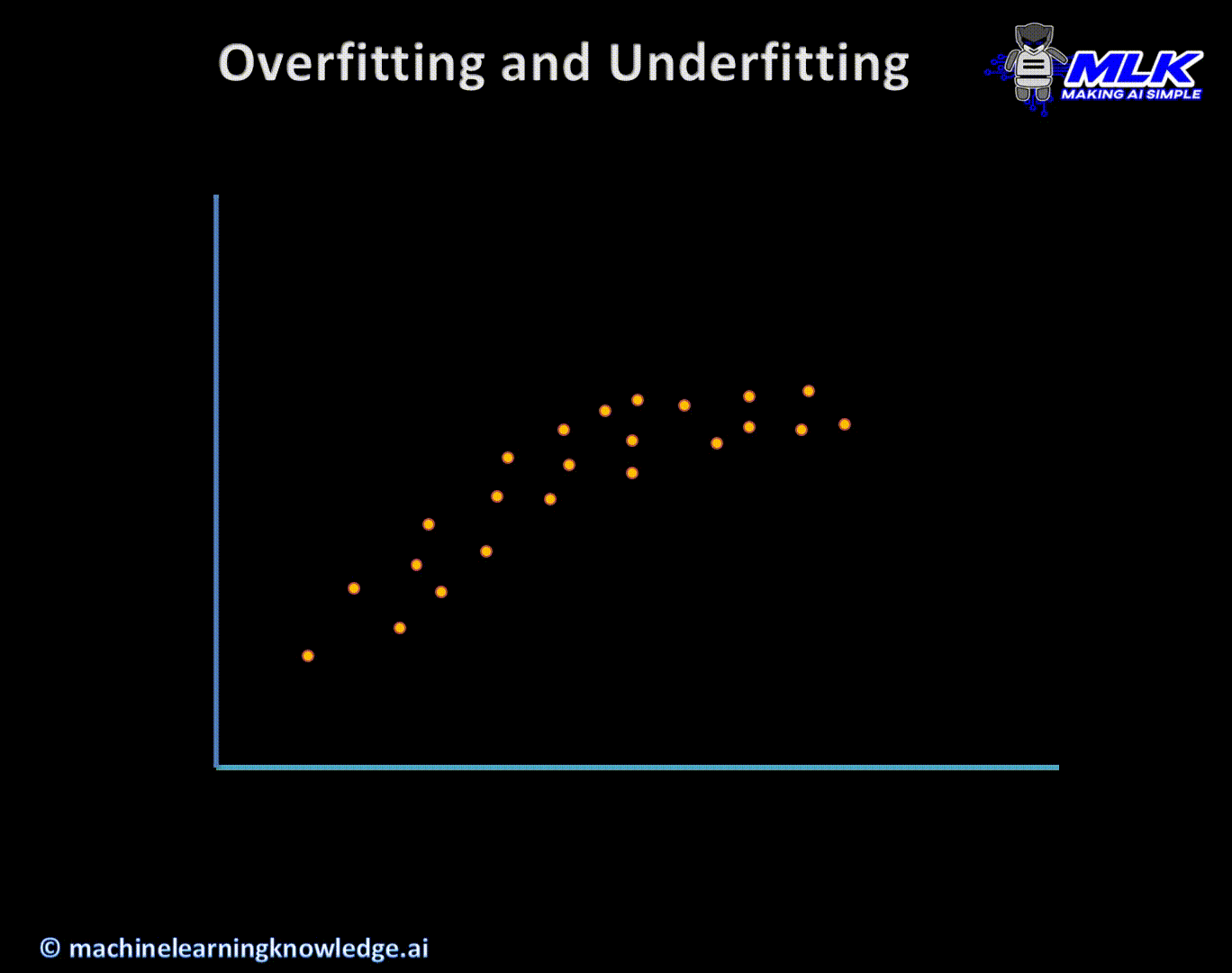## “K-means Clustering” in 200 words.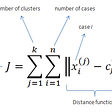## Here’s to partially random neural networks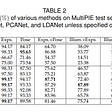## Machine learning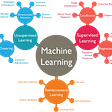## A Course Summary: Introduction to Machine Learning by Andrew Ng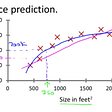## Cross validation in Data Science## Supervised and Unsupervised Machine Learning Algorithms